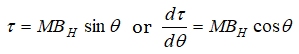Rate of change of torque  with deflections maximum for a magnet suspended freely in a uniform magnetic field of induction B, when

(a) $\theta ={0}^{o}$                        (b) $\theta ={45}^{o}$

(c) $\theta ={60}^{o}$                      (d) $\theta ={90}^{o}$

Concept Videos :-

#3 | Magnetic Dipole Moment & Field due to Bar Magnet
#4 | Superposition of Magnetic Field due to Bar Magnet
#5 | Field due to Short Bar Magnet
#6 | Torque Acting on Bar Magnet in Uniform Field
#7 | Work Done in Rotating Dipole in Field & PE
#8 | Oscillations of Bar Magnet in Uniform Field
#9 | Solenoid as Equivalent Bar Magnet

Concept Questions :-

Bar magnetThis will be maximum when, $\theta ={0}^{o}$

Difficulty Level:

• 30%
• 12%
• 8%
• 52%